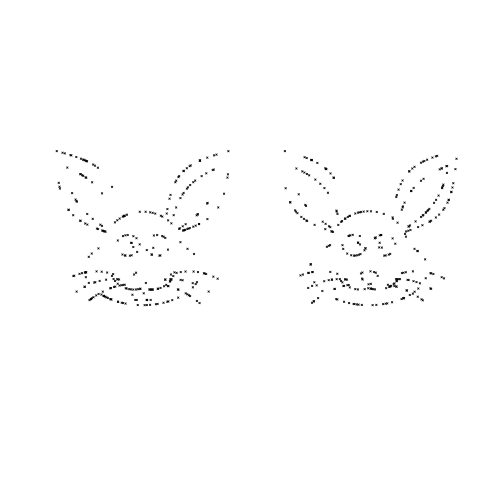# Automatically generated join-the-dots images

December 16, 2016
By

[This article was first published on The Shape of Code » R, and kindly contributed to R-bloggers]. (You can report issue about the content on this page here)
Want to share your content on R-bloggers? click here if you have a blog, or here if you don't.

It is interesting to try and figure out what picture emerges from a join-the-dots puzzle (connect-the-dots in some parts of the world). Let’s have a go at some lightweight automatic generation such a puzzle (some heavy-weight techniques).

If an image is available, expressed as an boolean matrix, R’s `sample` function can be used to select a small percentage of the black points.

Taking the output of the following equation:

``` x=seq(-4.7, 4.7, by=0.002)   y1 = c(1,-.7,.5)*sqrt(c(1.3, 2,.3)^2 - x^2) - c(.6,1.5,1.75) # 3 y2 =0.6*sqrt(4 - x^2)-1.5/as.numeric(1.3 <= abs(x)) # 1 y3 = c(1,-1,1,-1,-1)*sqrt(c(.4,.4,.1,.1,.8)^2 -(abs(x)-c(.5,.5,.4,.4,.3))^2) - c(.6,.6,.6,.6,1.5) # 5 y4 =(c(.5,.5,1,.75)*tan(pi/c(4, 5, 4, 5)*(abs(x)-c(1.2,3,1.2,3)))+c(-.1,3.05, 0, 2.6))/ as.numeric(c(1.2,.8,1.2,1) <= abs(x) & abs(x) <= c(3,3, 2.7, 2.7)) # 4 y5 =(1.5*sqrt(x^2 +.04) + x^2 - 2.4) / as.numeric(abs(x) <= .3) # 1 y6 = (2*abs(abs(x)-.1) + 2*abs(abs(x)-.3)-3.1)/as.numeric(abs(x) <= .4) # 1 y7 =(-.3*(abs(x)-c(1.6,1,.4))^2 -c(1.6,1.9, 2.1))/ as.numeric(c(.9,.7,.6) <= abs(x) & abs(x) <= c(2.6, 2.3, 2)) # 3 ```

and sampling 300 of the 20,012 points we get images such as the following:A relatively large sample size is needed to reduce the possibility that a random selection fails to return any points within a significant area, but we do end up with many points clustered here and there.

``` library("plyr")   rab_points=adply(x, 1, function(X) data.frame(x=rep(X, 18), y=c( c(1, -0.7, 0.5)*sqrt(c(1.3, 2, 0.3)^2-X^2) - c(0.6, 1.5 ,1.75), 0.6*sqrt(4 - X^2)-1.5/as.numeric(1.3 <= abs(X)), c(1, -1, 1, -1, -1)*sqrt(c(0.4, 0.4, 0.1, 0.1, 0.8)^2-(abs(X)-c(0.5, 0.5, 0.4, 0.4, 0.3))^2) - c(0.6, 0.6, 0.6, 0.6, 1.5), (c(0.5, 0.5, 1, 0.75)*tan(pi/c(4, 5, 4, 5)*(abs(X)-c(1.2, 3, 1.2, 3)))+c(-0.1, 3.05, 0, 2.6))/ as.numeric(c(1.2, 0.8, 1.2, 1) <= abs(X) & abs(X) <= c(3,3, 2.7, 2.7)), (1.5*sqrt(X^2+0.04) + X^2 - 2.4) / as.numeric(abs(X) <= 0.3), (2*abs(abs(X)-0.1)+2*abs(abs(X)-0.3)-3.1)/as.numeric(abs(X) <= 0.4), (-0.3*(abs(X)-c(1.6, 1, 0.4))^2-c(1.6, 1.9, 2.1))/ as.numeric(c(0.9, 0.7, 0.6) <= abs(X) & abs(X) <= c(2.6, 2.3, 2)) ))) rab_points\$X1=NULL rb=subset(rab_points, (!is.na(x)) & (!is.na(y) & is.finite(y)))   x=sample.int(nrow(rb), 300) plot(rb\$x[x], rb\$y[x], bty="n", xaxt="n", yaxt="n", pch=4, cex=0.5, xlab="", ylab="") ```

A more uniform image can produced by removing all points less than a given distance from some selected set of points. In this case the point in the first element is chosen, everything close to it removed and the the processed repeated with the second element (still remaining) and so on.

``` rm_nearest=function(jp) { keep=((dot_im\$x[(jp+1):(jp+window_size)]-dot_im\$x[jp])^2+ (dot_im\$y[(jp+1):(jp+window_size)]-dot_im\$y[jp])^2) < min_dist keep=c(keep, TRUE) # make sure which has something to return return(jp+which(keep)) }   window_size=500 cur_jp=1 dot_im=rb   while (cur_jp <= nrow(dot_im)) { # min_dist=0.05+0.50*runif(window_size) min_dist=0.05+0.30*runif(1) dot_im=dot_im[-rm_nearest(cur_jp), ] cur_jp=cur_jp+1 }   plot(dot_im\$x, dot_im\$y, bty="n", xaxt="n", yaxt="n", pch=4, cex=0.5, xlab="", ylab="") ```

Since R supports vector operations I want to do everything without using loops or if-statements. Yes, there is a `while` loop :-(, alternative, simple, non-loop suggestions welcome.

Removing points with an average squared distance less than 0.3 and 0.5 we get (with around 135-155 points) the images:I was going to come up with a scheme for adding numbers, perhaps I will do this in another post.

Click for more equations generating images.

R-bloggers.com offers daily e-mail updates about R news and tutorials about learning R and many other topics. Click here if you're looking to post or find an R/data-science job.
Want to share your content on R-bloggers? click here if you have a blog, or here if you don't.